# Product

Multiply and divide scalars and nonscalars or multiply and invert matrices

• Library:
• Simulink / Commonly Used Blocks

HDL Coder / Commonly Used Blocks

HDL Coder / HDL Floating Point Operations

HDL Coder / Math Operations

•## Description

The Product block outputs the result of multiplying two inputs: two scalars, a scalar and a nonscalar, or two nonscalars that have the same dimensions. The default parameter values that specify this behavior are:

• Multiplication: `Element-wise(.*)`

• Number of inputs: `2`

This table shows the output of the Product block for example inputs using default block parameter values.

Inputs and BehaviorExample

Scalar X Scalar

Output the product of the two inputs.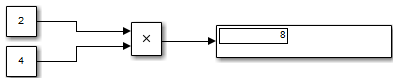Scalar X Nonscalar

Output a nonscalar having the same dimensions as the input nonscalar. Each element of the output nonscalar is the product of the input scalar and the corresponding element of the input nonscalar.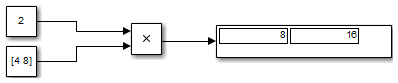Nonscalar X Nonscalar

Output a nonscalar having the same dimensions as the inputs. Each element of the output is the product of corresponding elements of the inputs.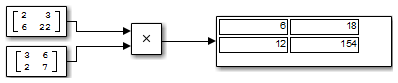The Divide and Product of Elements blocks are variants of the Product block.

The Product block (or the Divide block or Product of Elements block, if appropriately configured) can:

• Numerically multiply and divide any number of scalar, vector, or matrix inputs

• Perform matrix multiplication and division on any number of matrix inputs

The Product block performs scalar or matrix multiplication, depending on the value of the Multiplication parameter. The block accepts one or more inputs, depending on the Number of inputs parameter. The Number of inputs parameter also specifies the operation to perform on each input.

The Product block can input any combination of scalars, vectors, and matrices for which the operation to perform has a mathematically defined result. The block performs the specified operations on the inputs, then outputs the result.

The Product block has two modes: Element-wise mode, which processes nonscalar inputs element by element, and Matrix mode, which processes nonscalar inputs as matrices.

### Element-Wise Mode

When you set Multiplication to `Element-wise(.*)`, the Product block is in Element-wise mode, in which it operates on the individual numeric elements of any nonscalar inputs. The MATLAB® equivalent is the `.*` operator. In element-wise mode, the Product block can perform a variety of multiplication, division, and arithmetic inversion operations.

The value of the Number of inputs parameter controls both how many inputs exist and whether each is multiplied or divided to form the output. When the Product block is in element-wise mode and has only one input, it is functionally equivalent to a Product of Elements block. When the block has multiple inputs, any nonscalar inputs must have identical dimensions, and the block outputs a nonscalar with those dimensions. To calculate the output, the block first expands any scalar input to a nonscalar that has the same dimensions as the nonscalar inputs.

This table shows the output of the Product block for example inputs, using the indicated values for the Number of inputs parameter.

Parameter ValuesExamples

Number of inputs: `2`Number of inputs: `*/`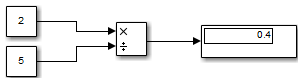Number of inputs: `/**/`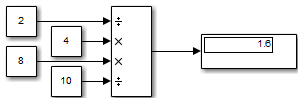Number of inputs:`**`Number of inputs: `*/*`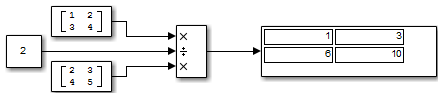### Matrix Mode

When the value of the Multiplication parameter is `Matrix(*)`, the Product block is in Matrix mode, in which it processes nonscalar inputs as matrices. The MATLAB equivalent is the `*` operator. In Matrix mode, the Product block can invert a single square matrix, or multiply and divide any number of matrices that have dimensions for which the result is mathematically defined.

The value of the Number of inputs parameter controls both how many inputs exist and whether each input matrix is multiplied or divided to form the output. The syntax of Number of inputs is the same as in element-wise mode. The difference between the modes is in the type of multiplication and division that occur.

### Expected Differences Between Simulation and Code Generation

For element-wise operations on complex floating-point inputs, simulation and code generation results might differ in near-overflow cases. Although complex numbers is selected and non-finite numbers is not selected on the Code Generation > Interface pane of the Configuration Parameters dialog box, the code generator does not emit special case code for intermediate overflows. This method improves the efficiency of embedded operations for the general case that does not include extreme values. If the inputs could include extreme values, you must manage these cases explicitly.

The generated code might not produce the same pattern of `NaN` and `inf` values as simulation when these values are mathematically meaningless. For example, if the simulation output contains a `NaN`, output from the generated code also contains a `NaN`, but not necessarily in the same place.

## Ports

### Input

expand all

First input to multiply or divide, provided as a scalar, vector, matrix, or N-D array.

Data Types: `half` | `single` | `double` | `int8` | `int16` | `int32` | `int64` | `uint8` | `uint16` | `uint32` | `uint64` | `Boolean` | `fixed point`

Nth input to multiply or divide, provided as a scalar, vector, matrix, or N-D array.

Data Types: `half` | `single` | `double` | `int8` | `int16` | `int32` | `int64` | `uint8` | `uint16` | `uint32` | `uint64` | `Boolean` | `fixed point`

Input signal to be multiplied with other inputs.

#### Dependencies

To enable one or more X ports, specify one or more `*` characters for the Number of inputs parameter.

Data Types: `half` | `single` | `double` | `int8` | `int16` | `int32` | `int64` | `uint8` | `uint16` | `uint32` | `uint64` | `Boolean` | `fixed point`

Input signal for division or inversion operations.

#### Dependencies

To enable one or more ÷ ports, specify one or more `/` characters for the Number of inputs parameter.

Data Types: `half` | `single` | `double` | `int8` | `int16` | `int32` | `int64` | `uint8` | `uint16` | `uint32` | `uint64` | `Boolean` | `fixed point`

### Output

expand all

Output computed by multiplying, dividing, or inverting inputs.

Data Types: `half` | `single` | `double` | `int8` | `int16` | `int32` | `int64` | `uint8` | `uint16` | `uint32` | `uint64` | `Boolean` | `fixed point`

## Parameters

expand all

### Main

Control two properties of the block:

• The number of input ports on the block

• Whether each input is multiplied or divided into the output

When you specify:

• `1` or `*` or `/`

The block has one input port. In element-wise mode, the block processes the input as described for the Product of Elements block. In matrix mode, if the parameter value is `1` or `*`, the block outputs the input value. If the value is `/`, the input must be a square matrix (including a scalar as a degenerate case) and the block outputs the matrix inverse. See Element-Wise Mode and Matrix Mode for more information.

• Integer value > 1

The block has the number of inputs given by the integer value. The inputs are multiplied together in element-wise mode or matrix mode, as specified by the Multiplication parameter. See Element-Wise Mode and Matrix Mode for more information.

• Unquoted string of two or more `*` and `/` characters

The block has the number of inputs given by the length of the character vector. Each input that corresponds to a `*` character is multiplied into the output. Each input that corresponds to a `/` character is divided into the output. The operations occur in element-wise mode or matrix mode, as specified by the Multiplication parameter. See Element-Wise Mode and Matrix Mode for more information.

#### Programmatic Use

 Block Parameter: `Inputs` Type: character vector Values: `'2' | '**' | '*/' | '*/*' | ...` Default: `'2'`

Specify whether the block performs `Element-wise(.*)` or `Matrix(*)` multiplication.

#### Programmatic Use

 Block Parameter: `Multiplication` Type: character vector Values: `'Element-wise(.*)' | 'Matrix(*)' ` Default: `'Element-wise(.*)'`

Specify the dimension to multiply over as `All dimensions`, or `Specified dimension`. When you select `Specified dimension`, you can specify the Dimension as `1` or `2`.

#### Dependencies

To enable this parameter, set Number of inputs to `*` and Multiplication to `Element-wise (.*)`.

#### Programmatic Use

 Block Parameter: `CollapseMode` Type: character vector Values: `'All dimensions' | 'Specified dimension'` Default: `'All dimensions'`

Specify the dimension to multiply over as an integer less than or equal to the number of dimensions of the input signal.

#### Dependencies

To enable this parameter, set:

• Number of inputs to `*`

• Multiplication to `Element-wise (.*)`

• Multiply over to `Specified dimension`

#### Programmatic Use

 Block Parameter: `CollapseDim` Type: character vector Values: `'1' | '2' | ...` Default: `'1'`

Specify the sample time as a value other than -1. For more information, see Specify Sample Time.

#### Dependencies

This parameter is not visible unless it is explicitly set to a value other than `-1`. To learn more, see Blocks for Which Sample Time Is Not Recommended.

#### Programmatic Use

 Block Parameter: `SampleTime` Type: character vector Values: scalar or vector Default: `'-1'`

### Signal Attributes

Specify if input signals must all have the same data type. If you enable this parameter, then an error occurs during simulation if the input signal types are different.

#### Programmatic Use

 Block Parameter: `InputSameDT` Type: character vector Values: `'off' | 'on'` Default: `'off'`

Lower value of the output range that Simulink® checks.

Simulink uses the minimum to perform:

Note

Output minimum does not saturate or clip the actual output signal. Use the Saturation block instead.

#### Programmatic Use

 Block Parameter: `OutMin` Type: character vector Values: `'[ ]'`| scalar Default: `'[ ]'`

Upper value of the output range that Simulink checks.

Simulink uses the maximum value to perform:

Note

Output maximum does not saturate or clip the actual output signal. Use the Saturation block instead.

#### Programmatic Use

 Block Parameter: `OutMax` Type: character vector Values: `'[ ]'`| scalar Default: `'[ ]'`

Choose the data type for the output. The type can be inherited, specified directly, or expressed as a data type object such as `Simulink.NumericType`. For more information, see Control Signal Data Types.

When you select an inherited option, the block behaves as follows:

• `Inherit: Inherit via internal rule` — Simulink chooses a data type to balance numerical accuracy, performance, and generated code size, while taking into account the properties of the embedded target hardware. If you change the embedded target settings, the data type selected by the internal rule might change. For example, if the block multiplies an input of type `int8` by a gain of `int16` and `ASIC/FPGA` is specified as the targeted hardware type, the output data type is `sfix24`. If ```Unspecified (assume 32-bit Generic)```, in other words, a generic 32-bit microprocessor, is specified as the target hardware, the output data type is `int32`. If none of the word lengths provided by the target microprocessor can accommodate the output range, Simulink software displays an error in the Diagnostic Viewer.

• `Inherit: Keep MSB`– Simulink chooses a data type that maintains the full range of the operation, then reduces the precision of the output to a size appropriate for the embedded target hardware.

Tip

For more efficient generated code, deselect the Saturate on integer overflow parameter.

This rule never produces overflows.

• `Inherit: Match scaling`– Simulink chooses a data type whose scaling matches the scaling of the input types. If the full range of the type does not fit on the embedded target hardware, the range is reduced yielding a type appropriate for the embedded target hardware. This rule can produce overflows. This rule does not support multiplication between complex signals

The `Inherit: Keep MSB` and `Inherit: Match scaling` rules do not support multiplication between complex signals or signals with non-zero bias. The rules support only multiplication and division (`'**'`, `'*/'`, `'/*'`) between two inputs, matrix multiplication of two inputs, and collapsing product of two elements of a vector.

It is not always possible for the software to optimize code efficiency and numerical accuracy at the same time. If the internal rule doesn’t meet your specific needs for numerical accuracy or performance, use one of the following options:

• Specify the output data type explicitly.

• Use the simple choice of ```Inherit: Same as input```.

• Explicitly specify a default data type such as `fixdt(1,32,16)` and then use the Fixed-Point Tool to propose data types for your model. For more information, see `fxptdlg` (Fixed-Point Designer).

• To specify your own inheritance rule, use ```Inherit: Inherit via back propagation``` and then use a Data Type Propagation block. Examples of how to use this block are available in the Signal Attributes library Data Type Propagation Examples block.

• ```Inherit: Inherit via back propagation``` — Use data type of the driving block.

• `Inherit: Same as first input` — Use data type of first input signal.

#### Dependencies

When input is a floating-point data type smaller than single precision, the ```Inherit: Inherit via internal rule``` output data type depends on the setting of the Inherit floating-point output type smaller than single precision configuration parameter. Data types are smaller than single precision when the number of bits needed to encode the data type is less than the 32 bits needed to encode the single-precision data type. For example, `half` and `int16` are smaller than single precision.

#### Programmatic Use

 Block Parameter: `OutDataTypeStr` Type: character vector Values: ```'Inherit: Inherit via internal rule``` | `'Inherit: Keep MSB'`| `'Inherit: Match scaling'`| `'Inherit: Same as first input'` | `'Inherit: Inherit via back propagation'` | `'double'` | `'single'` | `'half'` | `'int8'` | `'uint8'` | `'int16'` | `'uint16'` | `'int32'` | `'uint32'` | `'uint64'`|`'int64'`|`'fixdt(1,16)'` | `'fixdt(1,16,0)'` | `'fixdt(1,16,2^0,0)'` | ```''``` Default: ```'Inherit: Inherit via internal rule'```

Select this parameter to prevent the fixed-point tools from overriding the Output data type you specify on the block. For more information, see Use Lock Output Data Type Setting (Fixed-Point Designer).

#### Programmatic Use

 Block Parameter: `LockScale` Type: character vector Values: `'off' | 'on'` Default: `'off'`

Select the rounding mode for fixed-point operations. You can select:

`Ceiling`

Rounds positive and negative numbers toward positive infinity. Equivalent to the MATLAB `ceil` function.

`Convergent`

Rounds number to the nearest representable value. If a tie occurs, rounds to the nearest even integer. Equivalent to the Fixed-Point Designer™ `convergent` function.

`Floor`

Rounds positive and negative numbers toward negative infinity. Equivalent to the MATLAB `floor` function.

`Nearest`

Rounds number to the nearest representable value. If a tie occurs, rounds toward positive infinity. Equivalent to the Fixed-Point Designer `nearest` function.

`Round`

Rounds number to the nearest representable value. If a tie occurs, rounds positive numbers toward positive infinity and rounds negative numbers toward negative infinity. Equivalent to the Fixed-Point Designer `round` function.

`Simplest`

Chooses between rounding toward floor and rounding toward zero to generate rounding code that is as efficient as possible.

`Zero`

Rounds number toward zero. Equivalent to the MATLAB `fix` function.

Block parameters always round to the nearest representable value. To control the rounding of a block parameter, enter an expression using a MATLAB rounding function into the mask field.

#### Programmatic Use

 Block Parameter: `RndMeth` Type: character vector Values: ```'Ceiling' | 'Convergent' | 'Floor' | 'Nearest' | 'Round' | 'Simplest' | 'Zero'``` Default: `'Floor'`

Specify whether overflows saturate or wrap.

ActionRationaleImpact on OverflowsExample

Select this check box (`on`).

Your model has possible overflow, and you want explicit saturation protection in the generated code.

Overflows saturate to either the minimum or maximum value that the data type can represent.

The maximum value that the `int8` (signed, 8-bit integer) data type can represent is 127. Any block operation result greater than this maximum value causes overflow of the 8-bit integer. With the check box selected, the block output saturates at 127. Similarly, the block output saturates at a minimum output value of -128.

Do not select this check box (`off`).

You want to optimize efficiency of your generated code.

You want to avoid overspecifying how a block handles out-of-range signals. For more information, see Troubleshoot Signal Range Errors.

Overflows wrap to the appropriate value that is representable by the data type.

The maximum value that the `int8` (signed, 8-bit integer) data type can represent is 127. Any block operation result greater than this maximum value causes overflow of the 8-bit integer. With the check box cleared, the software interprets the overflow-causing value as `int8`, which can produce an unintended result. For example, a block result of 130 (binary 1000 0010) expressed as `int8`, is -126.

When you select this check box, saturation applies to every internal operation on the block, not just the output, or result. Usually, the code generation process can detect when overflow is not possible. In this case, the code generator does not produce saturation code.

#### Programmatic Use

 Block Parameter: `SaturateOnIntegerOverflow` Type: character vector Values: `'off' | 'on'` Default: `'off'`

## Block Characteristics

 Data Types `Boolean` | `double` | `fixed point` | `half` | `integer` | `single` Direct Feedthrough `yes` Multidimensional Signals `yes` Variable-Size Signals `yes` Zero-Crossing Detection `no`